Home » Knowledge Base » General » Materials » Radial Return

The most popular method for integrating the plasticity equations for isotropic von Mises plasticity is radial return, developed by Wilkins for HEMP and Maenchen and Sack for TENSOR, two finite difference codes developed at Lawrence Livermore National Laboratory.

It has been generalized to arbitrary convex yield surfaces and yield stress functions, although the return portion of the algorithm is no longer radial. Conceptually, the idea is quite simple: first the stress is updated assuming that the response is elastic, then if it is outside the yield surface, the stress is projected on to the closest point of the yield surface (Figure 1). If the material is perfectly plastic, the yield surface is constant, but if h > 0, the yield surface expands during the plastic flow, and the stress is projected on the expanded yield surface. The data available at the beginning of the radial return update is the previous stress, equivalent plastic strain, and increment in the strain across the time step, ε = t ε’. By the end of the radial return procedure, the stress and equivalent plastic strain have been updated. The superscripts n and n + 1 are dropped to simplify the notation in this section, and to make the equations in this section look as close to their actual appearance within a computer code as possible, where the old values are overwritten by the new values.

The trial stress, σ^Tr, is the deviatoric part of the stress calculated assuming that the response is elastic,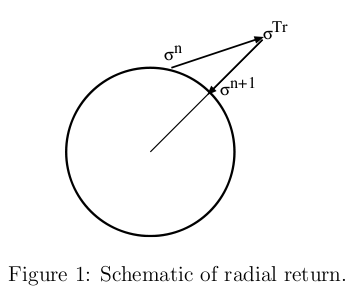but since the pressure is independent of the plastic flow, its update is the same regardless of whether or not there is plastic flow,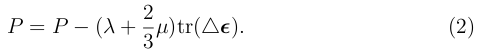The equivalent trial stress is evaluated,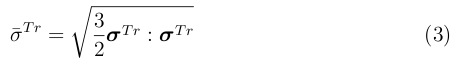and if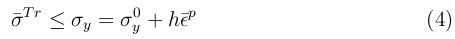then the response is elastic, and the stress at the end of the time step is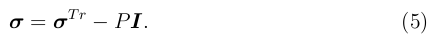When Equation 4 isn’t satisfied, there is plastic flow, and the increment in the equivalent plastic strain must be calculated. The closest point of the yield surface to the trial stress lies along the line from the origin to the trial stress, and therefore the final deviatoric stress will be proportional to the trial stress, σ ‘ = α σ^Tr. Regardless of the proportionality constant, the normal direction is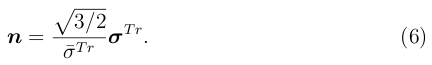The increment in equivalent plastic strain is calculated by requiring that the final equivalent stress equals the final yield stress,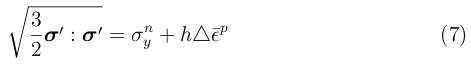where σy^n = hε^p and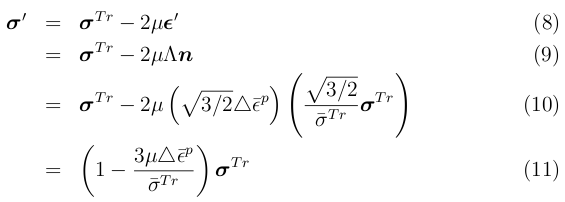Substituting in the last equation into Equation 7 results in a linear equation for the increment in equivalent plastic strain,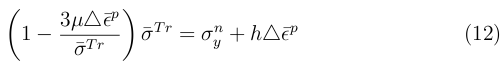so that, finally,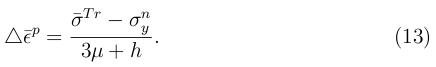Once the increment in equivalent plastic strain is calculated, the proportionality factor is calculated and the trial stress is scaled back to the yield surface. The stress update is completed by adding the deviatoric and mean stress, −P .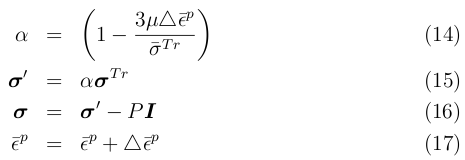djb 2005